Anzeige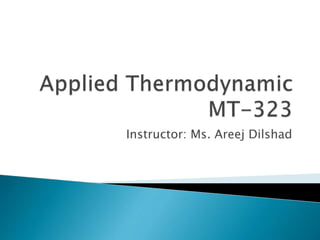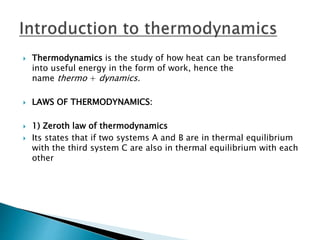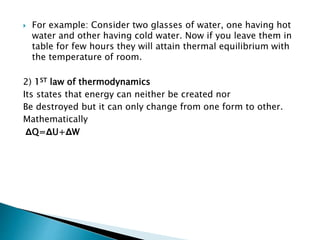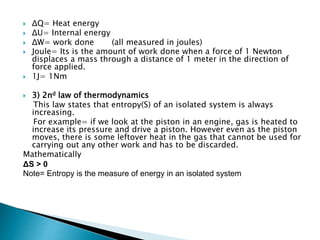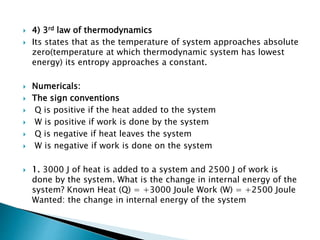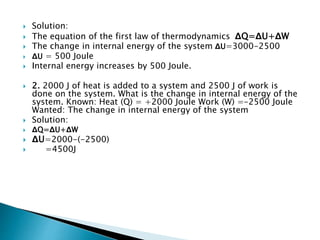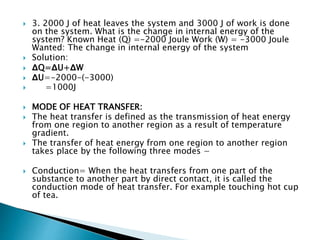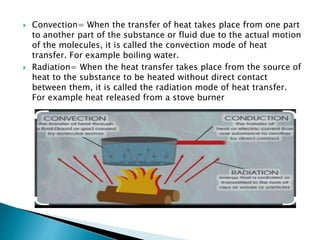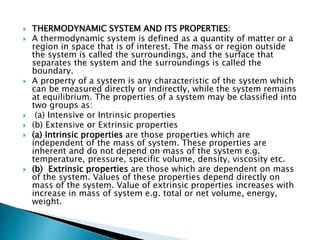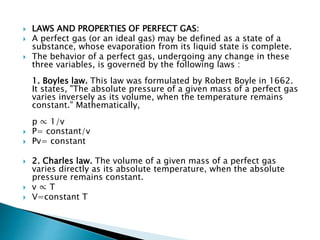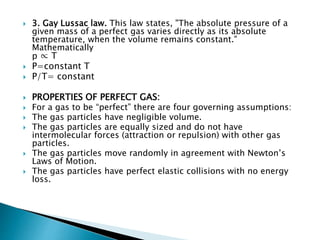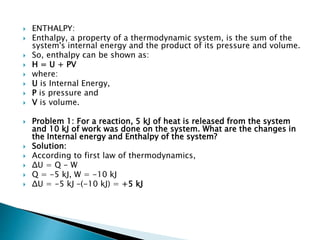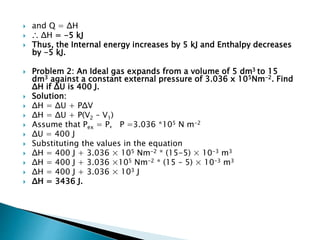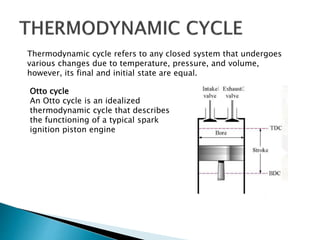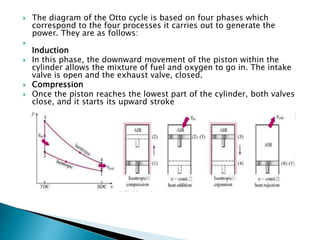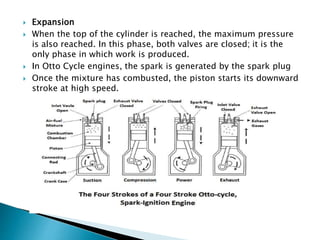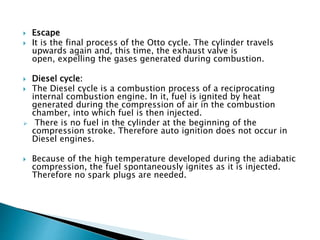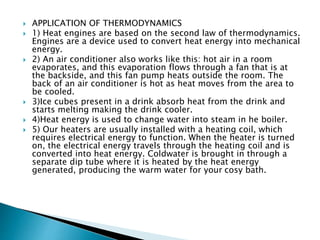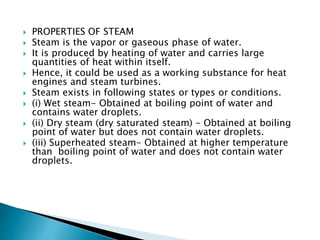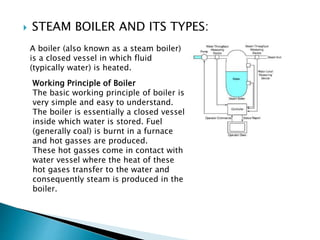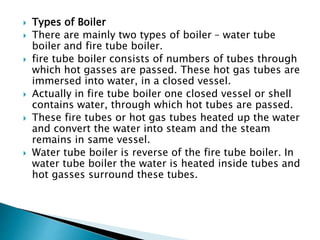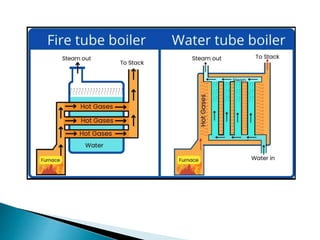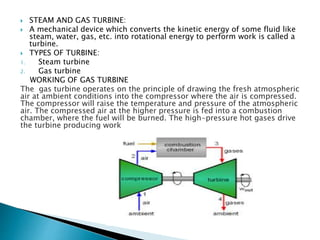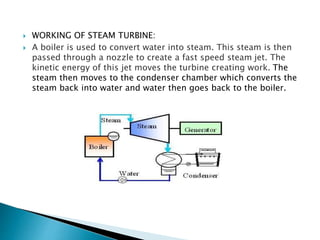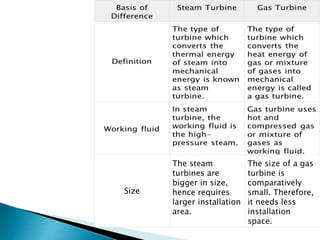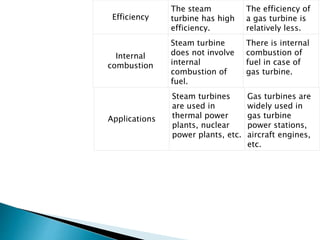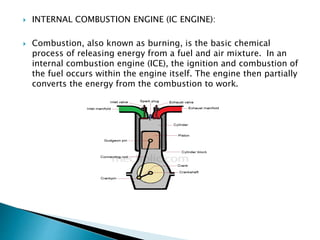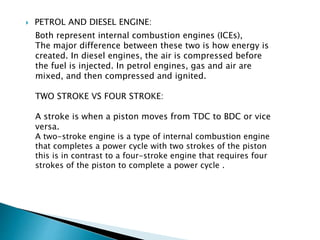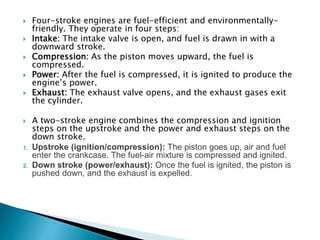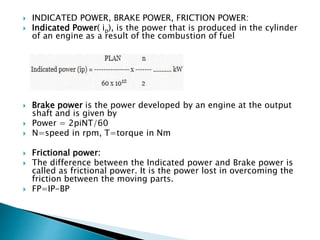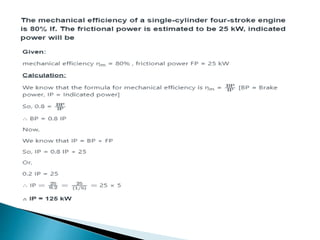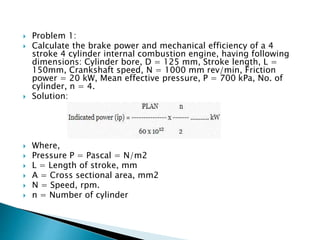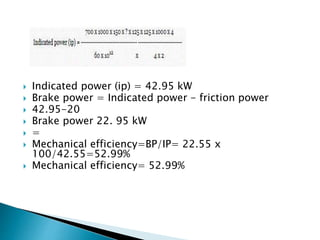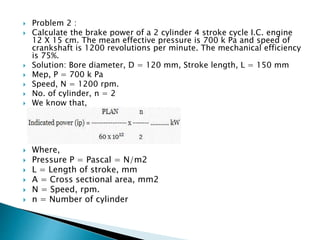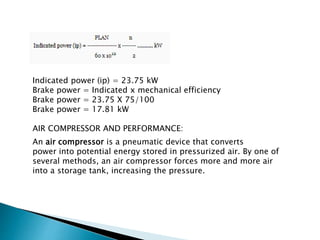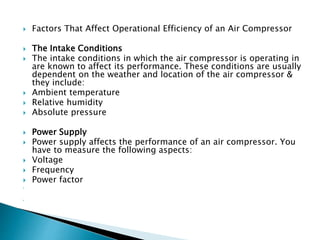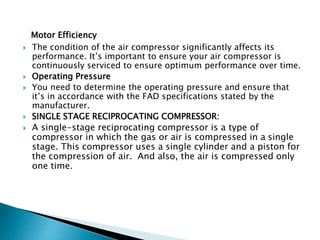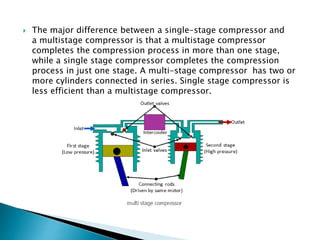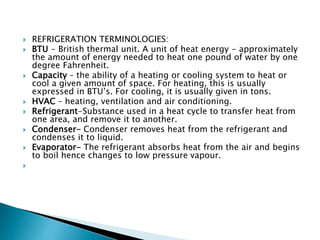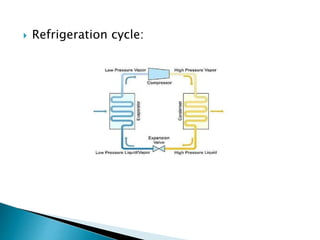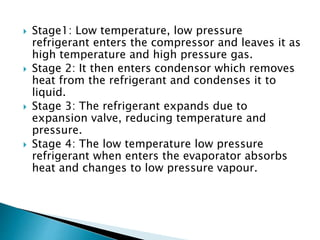1 von 41
Anzeige

### Applied Thermodynamic (1).pptx

2.  Thermodynamics is the study of how heat can be transformed into useful energy in the form of work, hence the name thermo + dynamics.  LAWS OF THERMODYNAMICS:  1) Zeroth law of thermodynamics  Its states that if two systems A and B are in thermal equilibrium with the third system C are also in thermal equilibrium with each other
3.  For example: Consider two glasses of water, one having hot water and other having cold water. Now if you leave them in table for few hours they will attain thermal equilibrium with the temperature of room. 2) 1ST law of thermodynamics Its states that energy can neither be created nor Be destroyed but it can only change from one form to other. Mathematically ΔQ=ΔU+ΔW
4.  ΔQ= Heat energy  ΔU= Internal energy  ΔW= work done (all measured in joules)  Joule= Its is the amount of work done when a force of 1 Newton displaces a mass through a distance of 1 meter in the direction of force applied.  1J= 1Nm  3) 2nd law of thermodynamics This law states that entropy(S) of an isolated system is always increasing. For example= if we look at the piston in an engine, gas is heated to increase its pressure and drive a piston. However even as the piston moves, there is some leftover heat in the gas that cannot be used for carrying out any other work and has to be discarded. Mathematically ΔS > 0 Note= Entropy is the measure of energy in an isolated system
5.  4) 3rd law of thermodynamics  Its states that as the temperature of system approaches absolute zero(temperature at which thermodynamic system has lowest energy) its entropy approaches a constant.  Numericals:  The sign conventions  Q is positive if the heat added to the system  W is positive if work is done by the system  Q is negative if heat leaves the system  W is negative if work is done on the system  1. 3000 J of heat is added to a system and 2500 J of work is done by the system. What is the change in internal energy of the system? Known Heat (Q) = +3000 Joule Work (W) = +2500 Joule Wanted: the change in internal energy of the system
6.  Solution:  The equation of the first law of thermodynamics ΔQ=ΔU+ΔW  The change in internal energy of the system ΔU=3000-2500  ΔU = 500 Joule  Internal energy increases by 500 Joule.  2. 2000 J of heat is added to a system and 2500 J of work is done on the system. What is the change in internal energy of the system. Known: Heat (Q) = +2000 Joule Work (W) =-2500 Joule Wanted: The change in internal energy of the system  Solution:  ΔQ=ΔU+ΔW  ΔU=2000-(-2500)  =4500J
7.  3. 2000 J of heat leaves the system and 3000 J of work is done on the system. What is the change in internal energy of the system? Known Heat (Q) =-2000 Joule Work (W) = -3000 Joule Wanted: The change in internal energy of the system  Solution:  ΔQ=ΔU+ΔW  ΔU=-2000-(-3000)  =1000J  MODE OF HEAT TRANSFER:  The heat transfer is defined as the transmission of heat energy from one region to another region as a result of temperature gradient.  The transfer of heat energy from one region to another region takes place by the following three modes −  Conduction= When the heat transfers from one part of the substance to another part by direct contact, it is called the conduction mode of heat transfer. For example touching hot cup of tea.
8.  Convection= When the transfer of heat takes place from one part to another part of the substance or fluid due to the actual motion of the molecules, it is called the convection mode of heat transfer. For example boiling water.  Radiation= When the heat transfer takes place from the source of heat to the substance to be heated without direct contact between them, it is called the radiation mode of heat transfer. For example heat released from a stove burner
9.  THERMODYNAMIC SYSTEM AND ITS PROPERTIES:  A thermodynamic system is defined as a quantity of matter or a region in space that is of interest. The mass or region outside the system is called the surroundings, and the surface that separates the system and the surroundings is called the boundary.  A property of a system is any characteristic of the system which can be measured directly or indirectly, while the system remains at equilibrium. The properties of a system may be classified into two groups as:  (a) Intensive or Intrinsic properties  (b) Extensive or Extrinsic properties  (a) Intrinsic properties are those properties which are independent of the mass of system. These properties are inherent and do not depend on mass of the system e.g. temperature, pressure, specific volume, density, viscosity etc.  (b) Extrinsic properties are those which are dependent on mass of the system. Values of these properties depend directly on mass of the system. Value of extrinsic properties increases with increase in mass of system e.g. total or net volume, energy, weight.
10.  LAWS AND PROPERTIES OF PERFECT GAS:  A perfect gas (or an ideal gas) may be defined as a state of a substance, whose evaporation from its liquid state is complete.  The behavior of a perfect gas, undergoing any change in these three variables, is governed by the following laws : 1. Boyles law. This law was formulated by Robert Boyle in 1662. It states, "The absolute pressure of a given mass of a perfect gas varies inversely as its volume, when the temperature remains constant." Mathematically, p ∝ 1/v  P= constant/v  Pv= constant  2. Charles law. The volume of a given mass of a perfect gas varies directly as its absolute temperature, when the absolute pressure remains constant.  v ∝ T  V=constant T
11.  3. Gay Lussac law. This law states, "The absolute pressure of a given mass of a perfect gas varies directly as its absolute temperature, when the volume remains constant." Mathematically p ∝ T  P=constant T  P/T= constant  PROPERTIES OF PERFECT GAS:  For a gas to be “perfect” there are four governing assumptions:  The gas particles have negligible volume.  The gas particles are equally sized and do not have intermolecular forces (attraction or repulsion) with other gas particles.  The gas particles move randomly in agreement with Newton’s Laws of Motion.  The gas particles have perfect elastic collisions with no energy loss.
12.  ENTHALPY:  Enthalpy, a property of a thermodynamic system, is the sum of the system's internal energy and the product of its pressure and volume.  So, enthalpy can be shown as:  H = U + PV  where:  U is Internal Energy,  P is pressure and  V is volume.  Problem 1: For a reaction, 5 kJ of heat is released from the system and 10 kJ of work was done on the system. What are the changes in the Internal energy and Enthalpy of the system?  Solution:  According to first law of thermodynamics,  ΔU = Q - W  Q = -5 kJ, W = -10 kJ  ΔU = -5 kJ –(-10 kJ) = +5 kJ
13.  and Q = ΔH  ∴ ΔH = -5 kJ  Thus, the Internal energy increases by 5 kJ and Enthalpy decreases by -5 kJ.  Problem 2: An Ideal gas expands from a volume of 5 dm3 to 15 dm3 against a constant external pressure of 3.036 x 105Nm-2. Find ΔH if ΔU is 400 J.  Solution:  ΔH = ΔU + PΔV  ΔH = ΔU + P(V2 – V1)  Assume that Pex = P, P =3.036 *105 N m-2  ΔU = 400 J  Substituting the values in the equation  ΔH = 400 J + 3.036 × 105 Nm-2 * (15-5) × 10-3 m3  ΔH = 400 J + 3.036 ×105 Nm-2 * (15 – 5) × 10-3 m3  ΔH = 400 J + 3.036 × 103 J  ΔH = 3436 J.
14. Thermodynamic cycle refers to any closed system that undergoes various changes due to temperature, pressure, and volume, however, its final and initial state are equal. Otto cycle An Otto cycle is an idealized thermodynamic cycle that describes the functioning of a typical spark ignition piston engine
15.  The diagram of the Otto cycle is based on four phases which correspond to the four processes it carries out to generate the power. They are as follows:  Induction  In this phase, the downward movement of the piston within the cylinder allows the mixture of fuel and oxygen to go in. The intake valve is open and the exhaust valve, closed.  Compression  Once the piston reaches the lowest part of the cylinder, both valves close, and it starts its upward stroke
16.  Expansion  When the top of the cylinder is reached, the maximum pressure is also reached. In this phase, both valves are closed; it is the only phase in which work is produced.  In Otto Cycle engines, the spark is generated by the spark plug  Once the mixture has combusted, the piston starts its downward stroke at high speed.
17.  Escape  It is the final process of the Otto cycle. The cylinder travels upwards again and, this time, the exhaust valve is open, expelling the gases generated during combustion.  Diesel cycle:  The Diesel cycle is a combustion process of a reciprocating internal combustion engine. In it, fuel is ignited by heat generated during the compression of air in the combustion chamber, into which fuel is then injected.  There is no fuel in the cylinder at the beginning of the compression stroke. Therefore auto ignition does not occur in Diesel engines.  Because of the high temperature developed during the adiabatic compression, the fuel spontaneously ignites as it is injected. Therefore no spark plugs are needed.
18.  APPLICATION OF THERMODYNAMICS  1) Heat engines are based on the second law of thermodynamics. Engines are a device used to convert heat energy into mechanical energy.  2) An air conditioner also works like this: hot air in a room evaporates, and this evaporation flows through a fan that is at the backside, and this fan pump heats outside the room. The back of an air conditioner is hot as heat moves from the area to be cooled.  3)Ice cubes present in a drink absorb heat from the drink and starts melting making the drink cooler.  4)Heat energy is used to change water into steam in he boiler.  5) Our heaters are usually installed with a heating coil, which requires electrical energy to function. When the heater is turned on, the electrical energy travels through the heating coil and is converted into heat energy. Coldwater is brought in through a separate dip tube where it is heated by the heat energy generated, producing the warm water for your cosy bath.
19.  PROPERTIES OF STEAM  Steam is the vapor or gaseous phase of water.  It is produced by heating of water and carries large quantities of heat within itself.  Hence, it could be used as a working substance for heat engines and steam turbines.  Steam exists in following states or types or conditions.  (i) Wet steam- Obtained at boiling point of water and contains water droplets.  (ii) Dry steam (dry saturated steam) - Obtained at boiling point of water but does not contain water droplets.  (iii) Superheated steam- Obtained at higher temperature than boiling point of water and does not contain water droplets.
20.  STEAM BOILER AND ITS TYPES: A boiler (also known as a steam boiler) is a closed vessel in which fluid (typically water) is heated. Working Principle of Boiler The basic working principle of boiler is very simple and easy to understand. The boiler is essentially a closed vessel inside which water is stored. Fuel (generally coal) is burnt in a furnace and hot gasses are produced. These hot gasses come in contact with water vessel where the heat of these hot gases transfer to the water and consequently steam is produced in the boiler.
21.  Types of Boiler  There are mainly two types of boiler – water tube boiler and fire tube boiler.  fire tube boiler consists of numbers of tubes through which hot gasses are passed. These hot gas tubes are immersed into water, in a closed vessel.  Actually in fire tube boiler one closed vessel or shell contains water, through which hot tubes are passed.  These fire tubes or hot gas tubes heated up the water and convert the water into steam and the steam remains in same vessel.  Water tube boiler is reverse of the fire tube boiler. In water tube boiler the water is heated inside tubes and hot gasses surround these tubes.
22.  STEAM AND GAS TURBINE:  A mechanical device which converts the kinetic energy of some fluid like steam, water, gas, etc. into rotational energy to perform work is called a turbine.  TYPES OF TURBINE: 1. Steam turbine 2. Gas turbine WORKING OF GAS TURBINE The gas turbine operates on the principle of drawing the fresh atmospheric air at ambient conditions into the compressor where the air is compressed. The compressor will raise the temperature and pressure of the atmospheric air. The compressed air at the higher pressure is fed into a combustion chamber, where the fuel will be burned. The high-pressure hot gases drive the turbine producing work
23.  WORKING OF STEAM TURBINE:  A boiler is used to convert water into steam. This steam is then passed through a nozzle to create a fast speed steam jet. The kinetic energy of this jet moves the turbine creating work. The steam then moves to the condenser chamber which converts the steam back into water and water then goes back to the boiler.
24. Size The steam turbines are bigger in size, hence requires larger installation area. The size of a gas turbine is comparatively small. Therefore, it needs less installation space.
25. Efficiency The steam turbine has high efficiency. The efficiency of a gas turbine is relatively less. Internal combustion Steam turbine does not involve internal combustion of fuel. There is internal combustion of fuel in case of gas turbine. Applications Steam turbines are used in thermal power plants, nuclear power plants, etc. Gas turbines are widely used in gas turbine power stations, aircraft engines, etc.
26.  INTERNAL COMBUSTION ENGINE (IC ENGINE):  Combustion, also known as burning, is the basic chemical process of releasing energy from a fuel and air mixture. In an internal combustion engine (ICE), the ignition and combustion of the fuel occurs within the engine itself. The engine then partially converts the energy from the combustion to work.
27.  PETROL AND DIESEL ENGINE: Both represent internal combustion engines (ICEs), The major difference between these two is how energy is created. In diesel engines, the air is compressed before the fuel is injected. In petrol engines, gas and air are mixed, and then compressed and ignited. TWO STROKE VS FOUR STROKE: A stroke is when a piston moves from TDC to BDC or vice versa. A two-stroke engine is a type of internal combustion engine that completes a power cycle with two strokes of the piston this is in contrast to a four-stroke engine that requires four strokes of the piston to complete a power cycle .
28.  Four-stroke engines are fuel-efficient and environmentally- friendly. They operate in four steps:  Intake: The intake valve is open, and fuel is drawn in with a downward stroke.  Compression: As the piston moves upward, the fuel is compressed.  Power: After the fuel is compressed, it is ignited to produce the engine’s power.  Exhaust: The exhaust valve opens, and the exhaust gases exit the cylinder.  A two-stroke engine combines the compression and ignition steps on the upstroke and the power and exhaust steps on the down stroke. 1. Upstroke (ignition/compression): The piston goes up, air and fuel enter the crankcase. The fuel-air mixture is compressed and ignited. 2. Down stroke (power/exhaust): Once the fuel is ignited, the piston is pushed down, and the exhaust is expelled.
29.  INDICATED POWER, BRAKE POWER, FRICTION POWER:  Indicated Power( ip), is the power that is produced in the cylinder of an engine as a result of the combustion of fuel  Brake power is the power developed by an engine at the output shaft and is given by  Power = 2piNT/60  N=speed in rpm, T=torque in Nm  Frictional power:  The difference between the Indicated power and Brake power is called as frictional power. It is the power lost in overcoming the friction between the moving parts.  FP=IP-BP
30.  Problem 1:  Calculate the brake power and mechanical efficiency of a 4 stroke 4 cylinder internal combustion engine, having following dimensions: Cylinder bore, D = 125 mm, Stroke length, L = 150mm, Crankshaft speed, N = 1000 mm rev/min, Friction power = 20 kW, Mean effective pressure, P = 700 kPa, No. of cylinder, n = 4.  Solution:  Where,  Pressure P = Pascal = N/m2  L = Length of stroke, mm  A = Cross sectional area, mm2  N = Speed, rpm.  n = Number of cylinder
31.  Indicated power (ip) = 42.95 kW  Brake power = Indicated power - friction power  42.95-20  Brake power 22. 95 kW  =  Mechanical efficiency=BP/IP= 22.55 x 100/42.55=52.99%  Mechanical efficiency= 52.99%
32.  Problem 2 :  Calculate the brake power of a 2 cylinder 4 stroke cycle I.C. engine 12 X 15 cm. The mean effective pressure is 700 k Pa and speed of crankshaft is 1200 revolutions per minute. The mechanical efficiency is 75%.  Solution: Bore diameter, D = 120 mm, Stroke length, L = 150 mm  Mep, P = 700 k Pa  Speed, N = 1200 rpm.  No. of cylinder, n = 2  We know that,  Where,  Pressure P = Pascal = N/m2  L = Length of stroke, mm  A = Cross sectional area, mm2  N = Speed, rpm.  n = Number of cylinder
33. Indicated power (ip) = 23.75 kW Brake power = Indicated x mechanical efficiency Brake power = 23.75 X 75/100 Brake power = 17.81 kW AIR COMPRESSOR AND PERFORMANCE: An air compressor is a pneumatic device that converts power into potential energy stored in pressurized air. By one of several methods, an air compressor forces more and more air into a storage tank, increasing the pressure.
34.  Factors That Affect Operational Efficiency of an Air Compressor  The Intake Conditions  The intake conditions in which the air compressor is operating in are known to affect its performance. These conditions are usually dependent on the weather and location of the air compressor & they include:  Ambient temperature  Relative humidity  Absolute pressure  Power Supply  Power supply affects the performance of an air compressor. You have to measure the following aspects:  Voltage  Frequency  Power factor  
35. Motor Efficiency  The condition of the air compressor significantly affects its performance. It’s important to ensure your air compressor is continuously serviced to ensure optimum performance over time.  Operating Pressure  You need to determine the operating pressure and ensure that it’s in accordance with the FAD specifications stated by the manufacturer.  SINGLE STAGE RECIPROCATING COMPRESSOR:  A single-stage reciprocating compressor is a type of compressor in which the gas or air is compressed in a single stage. This compressor uses a single cylinder and a piston for the compression of air. And also, the air is compressed only one time.
36.  The major difference between a single-stage compressor and a multistage compressor is that a multistage compressor completes the compression process in more than one stage, while a single stage compressor completes the compression process in just one stage. A multi-stage compressor has two or more cylinders connected in series. Single stage compressor is less efficient than a multistage compressor.
37.  REFRIGERATION TERMINOLOGIES:  BTU - British thermal unit. A unit of heat energy - approximately the amount of energy needed to heat one pound of water by one degree Fahrenheit.  Capacity – the ability of a heating or cooling system to heat or cool a given amount of space. For heating, this is usually expressed in BTU’s. For cooling, it is usually given in tons.  HVAC – heating, ventilation and air conditioning.  Refrigerant-Substance used in a heat cycle to transfer heat from one area, and remove it to another.  Condenser- Condenser removes heat from the refrigerant and condenses it to liquid.  Evaporator- The refrigerant absorbs heat from the air and begins to boil hence changes to low pressure vapour. 
38.  Refrigeration cycle:
39.  Stage1: Low temperature, low pressure refrigerant enters the compressor and leaves it as high temperature and high pressure gas.  Stage 2: It then enters condensor which removes heat from the refrigerant and condenses it to liquid.  Stage 3: The refrigerant expands due to expansion valve, reducing temperature and pressure.  Stage 4: The low temperature low pressure refrigerant when enters the evaporator absorbs heat and changes to low pressure vapour.
Anzeige